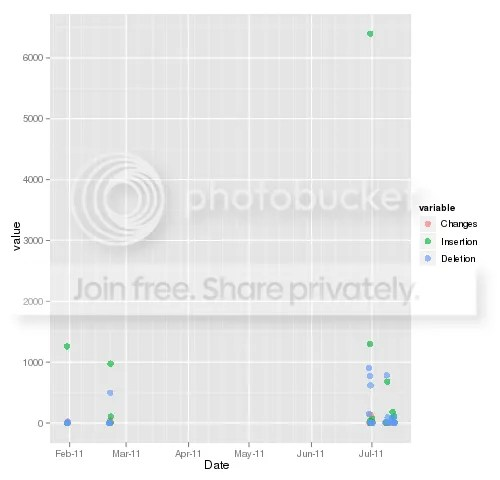Want to share your content on R-bloggers? click here if you have a blog, or here if you don't.

Here’s a funny story – friend of my, avid gamer at that time, was going downhill on a bicycle when wonderful idea flashed his mind: I need to save the current status… Just in case if I crash, I will start again from the top of the hill.

If you are a developer (quantitative or software), then you can use such marvelous feature. I use GitHub for my software and data mining or quantitative projects. Yesterday I came up with an idea to check my statistics of git commits. You can easily find ready to use software, but I was eager to extend my knowledge about git features and keep my machine clean.

I built two scripts – one is Linux shell script to get the data and another one is to plot the data in R.
getstats.sh:

 ```git log master --shortstat --pretty="format: %ai"| sed -e 's/\+[0-9]*/,/g'|sed ':a;N;\$!ba;s/ ,\n/,/g'| sed 's/ files changed//g'|sed 's/ insertions(,)//g'| sed 's/ deletions(-)//g' >gitstats.csv```

This part of the code: git log master –shortstat –pretty=”format: %ai” dumps all necessary data and the rest of the code makes it ready for R consumption. I found this page helpful, when I tried to format the dump.

gitStats.R:

?View Code RSPLUS
 ```require(ggplot2) require(xts) setwd('/home/git/Rproject/gitStats/') Sys.setenv(TZ="GMT") tmp=as.matrix(read.table('gitstats.csv',sep=',',header=FALSE)) commits=xts(cbind(as.double(tmp[,2]),as.double(tmp[,3]),as.double(tmp[,4])),order.by=as.POSIXct(strptime(tmp[,1],'%Y-%m-%d %H:%M:%S')))   colnames(commits)=c('Changes','Insertion','Deletion') tmp=data.frame(Date=as.Date(index(commits)),Changes=as.numeric(commits\$Changes),Insertion=as.numeric(commits\$Insertion),Deletion=as.numeric(commits\$Deletion)) tmp=melt(tmp,id.vars=c('Date')) png('gitStats.png',width=500) print(ggplot(tmp,aes(Date,value,color=variable))+geom_jitter(alpha=.65,size=3)) dev.off()   #############daily aggregated data############## factor=as.factor(format(index(commits),'%Y%m%d')) tmp=cbind(as.numeric(aggregate(commits\$Changes,factor,sum)),as.numeric(aggregate(commits\$Insertion,factor,sum)),as.numeric(aggregate(commits\$Deletion,factor,sum))) tmp=data.frame(unique(as.Date(index(commits))),tmp) colnames(tmp)=c('Date','Changes','Insertion','Deletion') tmp=melt(tmp,id.vars=c('Date')) png('gitStats2.png',width=500) print(ggplot(tmp,aes(Date,value,color=variable))+geom_jitter(alpha=.65,size=3)) dev.off()```

R script generates this nice plot below:What does it shows? It shows my activity in master repository. There is two projects – one was suspended in March and another one is under heavy development. As you can see, there was a lot of insertion when the last project was committed and since then numbers of insertion declined. I will come back, when I generate more data.
Do you track your git activity?

Source code